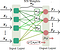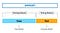Even if you are not a programmer, some things like these are popping up from time to time:

And what if it’s easy to get a pass behind the “neural scenes”? Our developer Oleg has collected the minimum to peep into the topic and, possibly, stay there.

Hi everyone, I’m padawan to neural network (NN) training and would like to share the basics about it — in short & sticking to the point.

Where NOT to start?

Of course, to run ahead of a train (as neural network is a quite big locomotive!), namely:

- walk through the materials like “make your own neural network in two hours”

- repeat the actions from the tutorials from elvis, Arthur Arnx, Johannes Rieke and others ( you’re getting the expected result, but without understanding what’s going on)

Easter egg: hearing my conversations with the monitor, a colleague gave me a bit of simple but cool advice — “Hey man, start with the basics”.

What is Neural Network?

Putting aside the practical part, I exhaled, took a cup of coffee and googled — what does a NN consist of? (well it works, educates, interacts, cooks scrambled eggs and plays tennis of course!)

After understanding the Perceptron and cheat sheet, I learned that:

a. there are many types of neural networks: a direct propagation network, a neural network of radial-basis functions, a Hopfield network, etc.

b. it’s worth choosing a “direct propagation neural network” as the most intuitive one:Fig. 1 — An example of a simple direct propagation network(Source — Gabor Melli’s Research Knowledge Base)

c. Neural network consists of layers with neurons and connections between them. The layers are divided into input, output and intermediate (hidden) ones. The number of neurons in the input layer corresponds to the amount of data supplied to the model for analysis/training.

Example: if the input data is an image of 28x28 pixels, then you need to have 784 neurons on the input layer — one for each pixel of the image. There should be as many neurons in the output layer as the input data processing variants we expect to receive. For example, if the model recognizes objects in images, the number of neurons in the output layer will be equal to the number of objects that the model can recognize in the image.

The number of hidden layers and neurons in them depends on the complexity and quantity of connections between the input and output data. The neurons between these layers are connected by synapses. Each synapse has its own weight.

Generally, that’s all about neural network components and their interaction, so let’s dive into how it is trained.

How it’s trained?

In order to have a neural network to be working with any input data, the given data needs to be converted to numeric values. Let’s go back to the example with the image. In the beginning, we make it black and white. Then each pixel is converted to a value between 0 and 1 depending on its brightness. Next, we fill in the first (input) layer of neurons with the input data. The neurons of the first layer transmit these values to the neurons of the next layer via synapses (connections). A neuron that receives a synapse value from another neuron will receive a value equal to:

“value from the sending neuron” x “weight of the synapse” = received value

But a neuron can receive signals from several synapses. To get the output value for the neuron, the activator functions are used. There are several types of activator functions (sigmoid, linear, step, ReLU, tahn). When the data transmitted to the input passes through all the neurons of the neural network and the connections between them in the neurons, we will get the result of the neural network in the output layer.

As I mentioned above, we will have as many neurons in the output layer as the results we are expecting to receive.

Example: let’s say the network will be used for recognition of numbers in the image, so the number of neurons should be equal to 10 — one for each digit from 0 to 9. Now we’re going to look at the value in each neuron of the output layer — these values will show the probability that one of the numbers has been found in the picture. Let’s say we got the following set of results for all 10 neurons: [0.13, 0.32, 0.14, 0.17, 0.12, 0.15, 0.1, 0.85, 0.14, 0.25]. Neuron number 8 has the biggest result. If during the training stage this neuron corresponded to the number 8, it means the model recognized an 8 in the picture :)

How to implement?
I tried to teach the network how to play tic-tac-toe.

For creating my first NN I chose Tensorflow, as it’s the most popular framework out there. Following the official documentation, I coded my first Neural Network:

STEP 1 — Code that creates the model’s layers:

hidden_1_layer = {‘f_fum’:n_nodes_hl1,
'weight':tf.Variable(tf.random_normal([cols_of_model, n_nodes_hl1])),
'bias':tf.Variable(tf.random_normal([n_nodes_hl1]))}
hidden_2_layer = {'f_fum':n_nodes_hl2,
'weight':tf.Variable(tf.random_normal([n_nodes_hl1, n_nodes_hl2])),
'bias':tf.Variable(tf.random_normal([n_nodes_hl2]))}
hidden_3_layer = {'f_fum':n_nodes_hl3,
'weight':tf.Variable(tf.random_normal([n_nodes_hl2, n_nodes_hl3])),
'bias':tf.Variable(tf.random_normal([n_nodes_hl3]))}
output_layer = {'f_fum':None,
'weight':tf.Variable(tf.random_normal([n_nodes_hl3, n_classes])),
'bias':tf.Variable(tf.random_normal([n_classes])),}

STEP 2 — Next, we’re going to need a function to initialize the neural network model.

Example:

def neural_network_model(data):
l1 = tf.nn.relu(l1)
l2 = tf.nn.relu(l2)
l3 = tf.nn.relu(l3)
output = tf.matmul(l3,output_layer['weight']) + output_layer['bias']
return output

STEP 3 — As well as a function for training and saving the trained model:

def train_neural_network(x):
prediction = neural_network_model(x)
cost = tf.reduce_mean( tf.nn.softmax_cross_entropy_with_logits(logits = prediction,labels=y) )
saver = tf.train.Saver()
with tf.Session() as sess:
sess.run(tf.global_variables_initializer())
train_x,train_y,test_x,test_y = work()
for epoch in range(hm_epochs):
c = list(zip(train_x, train_y))
random.shuffle(c)
train_x, train_y = zip(*c)
epoch_loss = 0
i=0
while i < len(train_x):
start = i
end = i+batch_size
batch_x = np.array(train_x[start:end])
batch_y = np.array(train_y[start:end])
_, c = sess.run([optimizer, cost], feed_dict={x: batch_x,
y: batch_y})
epoch_loss += c
i+=batch_size
if((epoch+1) % 500 == 0):
correct = tf.equal(tf.argmax(prediction, 1), tf.argmax(y, 1))
accuracy = tf.reduce_mean(tf.cast(correct, 'float'))
acc=accuracy.eval({x:test_x, y:test_y})
if epoch_loss == 0: break
correct = tf.equal(tf.argmax(prediction, 1), tf.argmax(y, 1))
accuracy = tf.reduce_mean(tf.cast(correct, 'float'))
acc=accuracy.eval({x:test_x, y:test_y})
save_path = saver.save(sess, "./modelNext/modelNext.ckpt")

STEP 4 — The training of a neural network model consists of feeding it two datasets: a training one and a testing one.Fig. 2 — Splitting the dataset into Training and Testing datasets (Source — Analytics Vidhya)

The training dataset contains data for processing as well as the correct processing results, and the testing one only contains data for processing. Based on the errors from working with the testing dataset, I changed the weights of synapses between neurons using the error backpropagation method. This method changes the weights of the synapses depending on the magnitude of the error made by the model.

STEP 5 — Lookup a tic-tac-toe dataset generator — any will do, for example here’s the one I used. And use it to generate a dataset:

I split the generated dataset into the training and testing datasets. After each training epoch, I corrected the weights of the synapses using the backpropagation method until I got a model which only played for a draw or a win.

STEP 6 — Create a function to use the trained model:

def use_neural_network(data):
prediction = neural_network_model(x)
saver = tf.train.Saver()
with tf.Session() as sess:
sess.run(tf.global_variables_initializer())
saver.restore(sess,"./modelNext/modelNext.ckpt")
for i in data:
result = (sess.run(tf.argmax(prediction.eval(feed_dict={x:[i]}),1)))

STEP 7 — Now we can create a simple Flask server and a client app (I used Angular).

STEP 8 — The client app will be sending the game board state to the server:

move(event) {
const cell = +event.target.id;
this.board[cell] = -1;
if (this.getWinner()) {
this.http
.post('http://0.0.0.0/api/tic', this.board, httpOptions)
.toPromise()
.then((res: any) => {
this.board[res] = 1;
this.getWinner();
});
}
}

STEP 9 — The server will feed the board state to the trained model, and it will return the best possible move:

def bestmove(input):
global graph
with graph.as_default():
data = (sess.run(tf.argmax(prediction.eval(session = sess,feed_dict={x:[input]}),1)))
return data
@app.route('/api/tic', methods=['POST'])
def tic_api():
data = request.get_json(force=True)
data = np.array(data)
data = data.tolist()
return jsonify(np.asscalar(bestmove(data)))

STEP 10 — Here it is, working, in all of its glory!